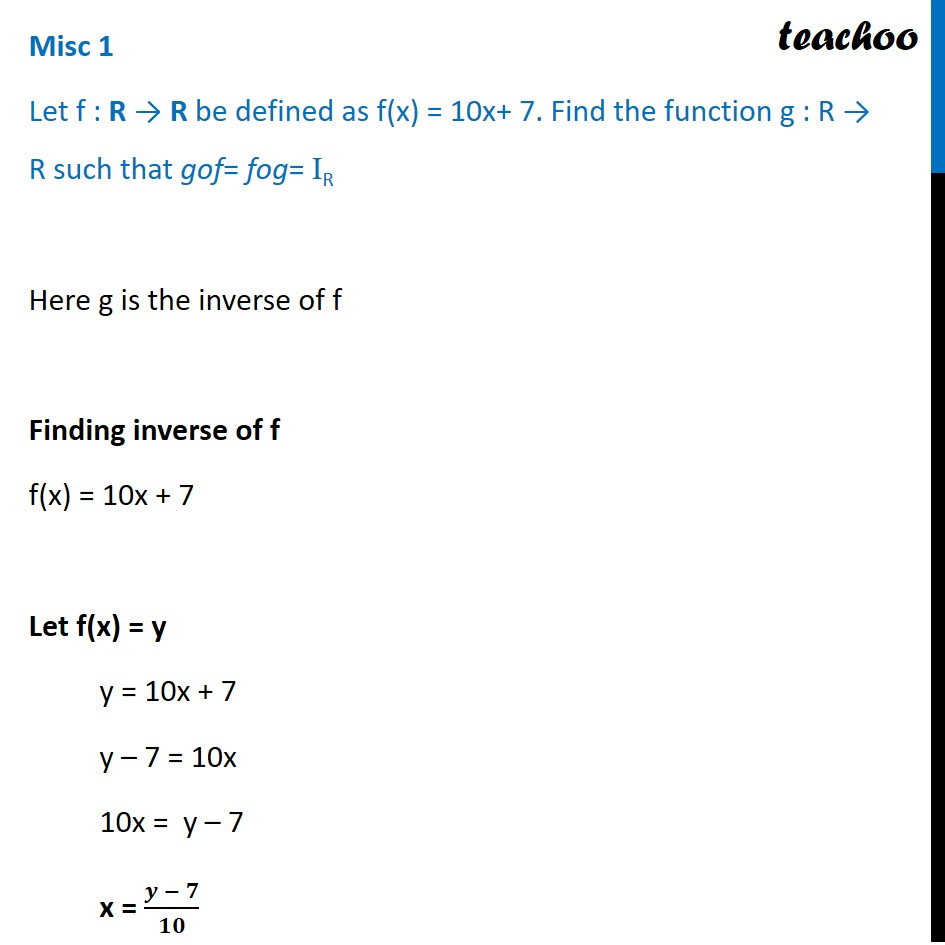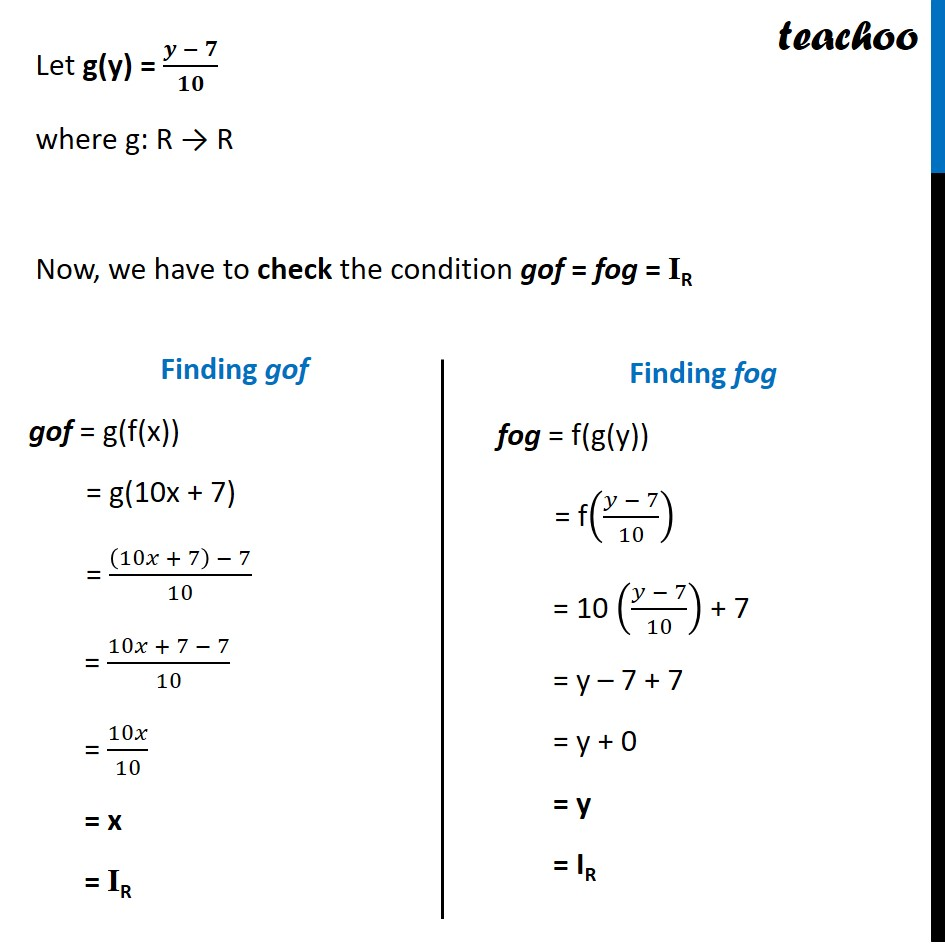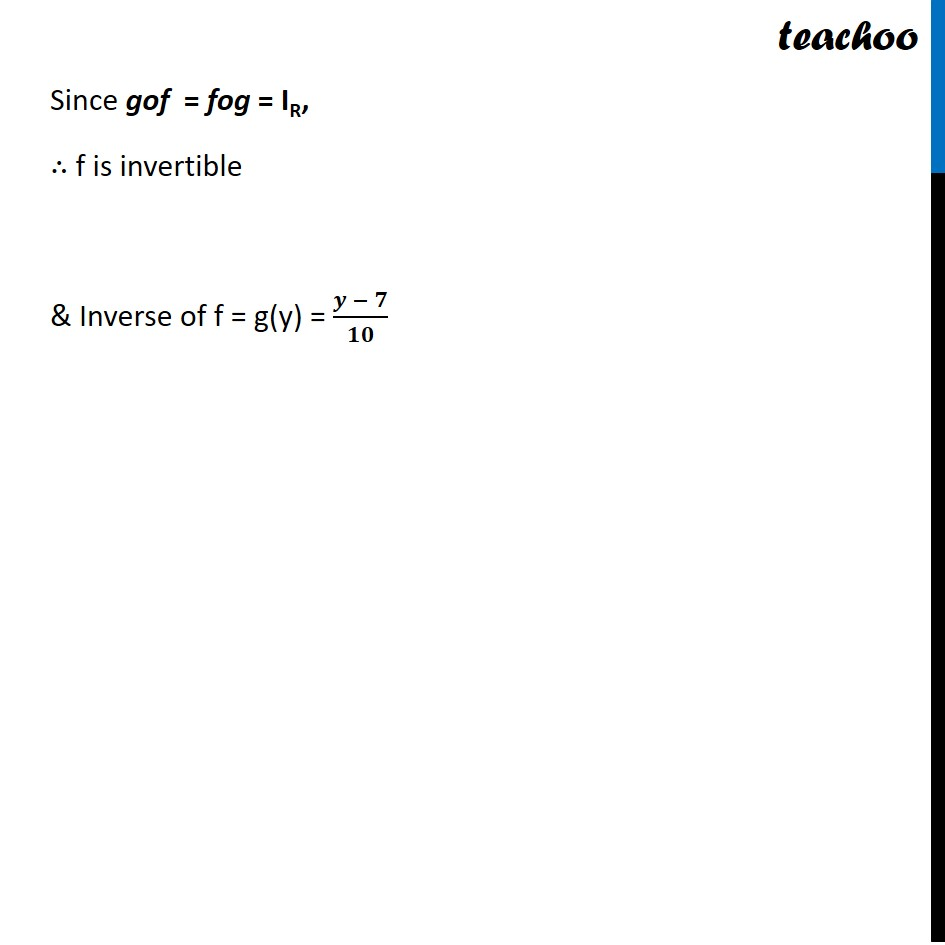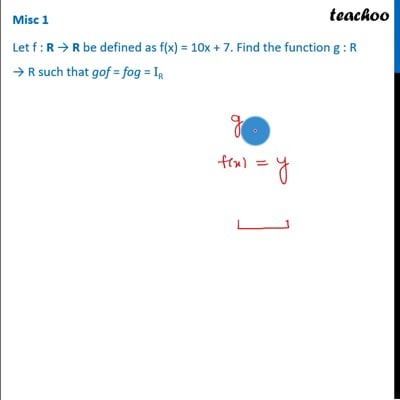Finding Inverse

Chapter 1 Class 12 Relation and Functions
Concept wiseThis video is only available for Teachoo black users

Introducing your new favourite teacher - Teachoo Black, at only ₹83 per month

### Transcript

Misc 1 Let f : R → R be defined as f(x) = 10x+ 7. Find the function g : R → R such that gof= fog= IR Here g is the inverse of f Finding inverse of f f(x) = 10x + 7 Let f(x) = y y = 10x + 7 y – 7 = 10x 10x = y – 7 x = (𝒚 − 𝟕)/𝟏𝟎 Let g(y) = (𝒚 − 𝟕)/𝟏𝟎 where g: R → R Now, we have to check the condition gof = fog = IR Finding gof gof = g(f(x)) = g(10x + 7) = ((10𝑥 + 7) − 7)/10 = (10𝑥 + 7 − 7)/10 = 10𝑥/10 = x = IR Finding fog fog = f(g(y)) = f((𝑦 − 7)/10) = 10 ((𝑦 − 7)/10) + 7 = y – 7 + 7 = y + 0 = y = IR Since gof = fog = IR, ∴ f is invertible & Inverse of f = g(y) = (𝒚 − 𝟕)/𝟏𝟎